Checkout JEE MAINS 2022 Question Paper Analysis : Checkout JEE MAINS 2022 Question Paper Analysis :

# I.E. Irodov Solutions on Laws of Conservation of Energy, Momentum, and Angular Momentum

Students can find the solutions to Problems In General Physics I.E. Irodov - Laws of Conservation of Energy, Momentum, and Angular Momentum on this page. When Physical Fundamentals of Mechanics is considered, Laws of Conservation of Energy, Momentum, and Angular Momentum is an important topic. Mechanics deals with motion and forces producing motion. The important topics include work and power of the force, increment of the kinetic energy of a particle, the relationship between the force of a field and the potential energy of a particle in the field, Law of momentum variation of a system, equation of motion of the system's centre of inertia, etc.

Revising these solutions will help students to easily score better ranks in the JEE exam and other competitive exams.

### I.E. Irodov Solutions on Laws of Conservation of Energy, Momentum, and Angular Momentum

1. A particle has shifted along some trajectory in the plane xy from point 1 whose radius vector r1 = i + 2j to point 2 with the radius vector r2 = 2i - 3j. During that time the particle experienced the action of certain forces, one of which being F = 3i + 4j. Find the work performed by the force F. (Here r1, r2 and F are given in SI units).

Solution:

1. As

$$\vec{F}$$
is constant so the sought work done

$$A=\vec{F}.\Delta \vec{r}= \vec{F}.(\vec{r}_{2}-\vec{r}_{1})$$

Or A =

$$(3\vec{i}+4\vec{j}).((2\vec{i}-3\vec{j})-(\vec{i}+2\vec{j}))$$

= 17 J

2. A locomotive of mass m starts moving so that its velocity varies according to the law v = a√s where a is a constant, and s is the distance covered. Find the total work performed by all the forces which are acting on the locomotive during the first t seconds after the beginning of motion.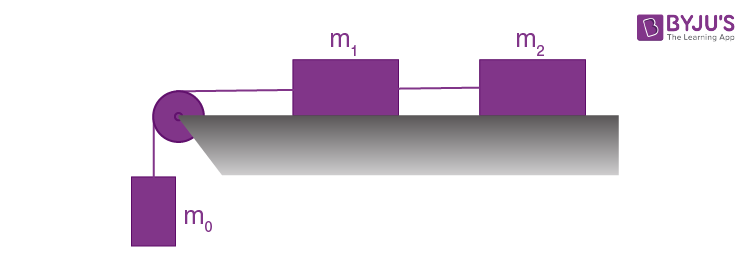Solution:

1. Differentiating v(s) with respect to time

dv/dt = a/2√s)ds/dt = (a/2√s)a√s

= a2/2

= w

(As locomotive is in unidirectional motion)

Hence force acting on the locomotive F = mw = ma2/2

Let, at v = 0 at t = 0 then the distance covered during the first t seconds

s = ½ wt2 = ½ (a2/2)t2 = a2t2/4

Hence the sought work, A = Fs = (ma2/2)(a2t2)/4 = ma4t2/8

3. The kinetic energy of a particle moving along a circle of radius R depends on the distance covered s as T = as2, where a is a constant. Find the force acting on the particle as a function of S.

Solution:

1. We have T = ½ mv2 = as2

Or v2 = 2as2/m (1)

Differentiating Eq.(1) with respect to time

2vwt = 4asv/m or wt = 2as/m (2)

Hence net acceleration of the particle

w = √(wt2 + wn2)

= √[(2as/m)2 + (2as2/mR)2]

= (2as/m)√(1 + (s/R))2

Hence the sought force, F = mw = 2as√(1 + (s/R)2)

4. A body of mass m was slowly hauled up the hill (Fig. 1.29) by a force F which at each point was directed along a tangent to the trajectory. Find the work performed by this force, if the height of the hill is h, the length of its base l, and the coefficient of friction k.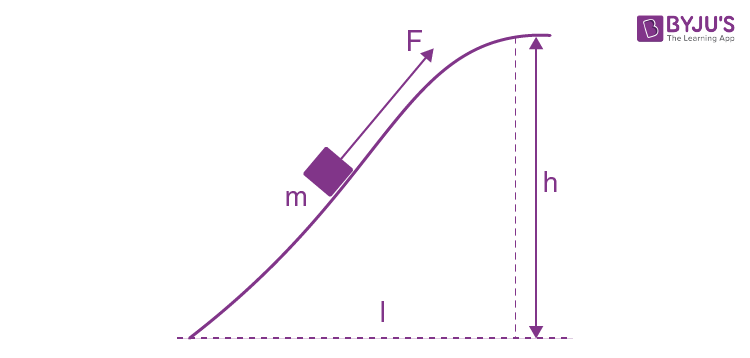Solution:

1. Let vector F makes an angle θ with the horizontal at any instant of time (Fig.). Newton’s second law in projection form along the direction of the force, gives : F = kmg cos θ + mg sin θ (because there is no acceleration of the body.)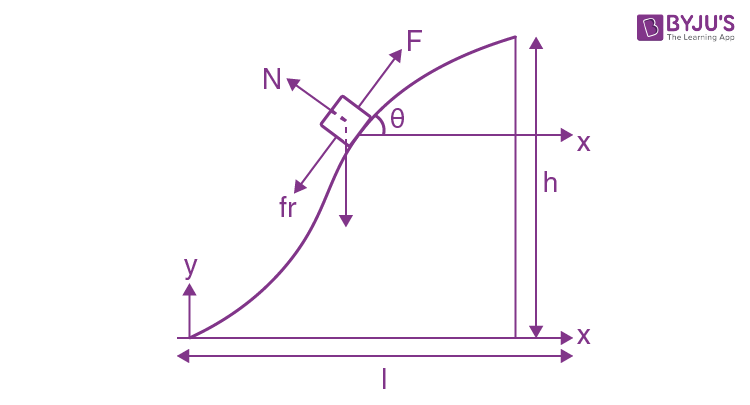As

$$\vec{F}$$
↑ ↑
$$d\vec{r}$$
the differential work done by the force
$$\vec{F}$$
,

dA =

$$\vec{F}.d\vec{r}= F\: ds$$

= kmg ds cos θ + mg ds sin θ

= kmg dx + mg dy

Hence

$$A = kmg\int_{0}^{l}dx + mg\int_{0}^{h}dy$$

= kmgl + mgh

= mg(kl + h)

5. A disc of mass m = 50 g slides with the zero initial velocity down an inclined plane set at an angle α = 300 to the horizontal; having traversed the distance l = 50 cm along the horizontal plane, the disc stops. Find the work performed by the friction forces over the whole distance, assuming the friction coefficient k = 0.15 for both inclined and horizontal planes.

Solution:

1. Let s be the distance covered by the disc along the incline, from the Eq. of increment of M.E. of the disc in the field of gravity :

M.E of the disc in the field of gravity: ∆T + ∆U = Afr

0 + (-mgs sin α) = -kmg cos α s - kmgl

Or s = kl/sin α - k cos α (1)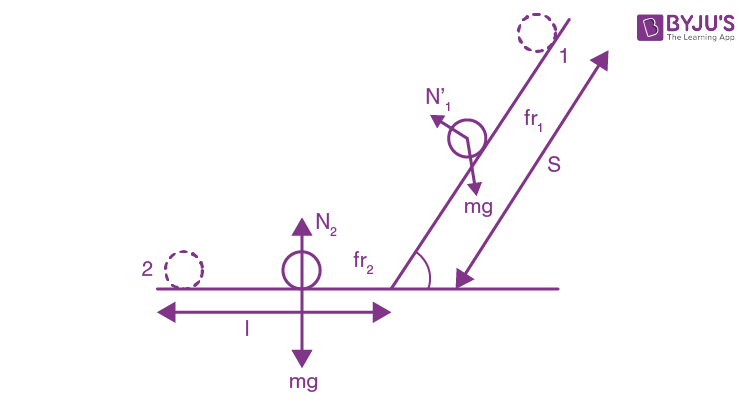Hence the sought work

Afr = -kmg (s cos α + l)

Afr = -klmg/(1 - k cot α) (using the eqn (1))

On putting the values Afr = -0.05J

6. Two bars of masses m1 and m2 connected by a non-deformed light spring rest on a horizontal plane. The coefficient of friction between the bars and the surface is equal to k. What minimum constant force has to be applied in the horizontal direction to the bar of mass m1 in order to shift the other bar?

Solution:

1. Let x be the compression in the spring when the bar m2 is about to shift. Therefore at this moment spring force on m2 is equal to the limiting friction between the bar m2 and horizontal floor. Hence

kx = k m2g [where k is the spring constant (say)] (1)

For the block m1 from work-energy theorem: A = ∆T = 0 for minimum force. (A here includes the work done in stretching the spring.)

So Fx - ½ kx2 - kmgx = 0

Or kx/2 = F - km1g (2)

From (1) and (2)

F = kg(m1 + m2/2)

7. A chain of mass m = 0.80 kg and length l = 1.5 m rests on a rough-surfaced table so that one of its ends hangs over the edge. The chain starts sliding off the table all by itself provided the overhanging part equals η = 1/3 of the chain length. What will be the total work performed by the friction forces acting on the chain by the moment it slides completely off the table?

Solution:

1. From the initial condition of the problem the limiting friction between the chain lying on the horizontal table equals the weight of the over hanging part of the chain, i.e

ληlg = kλ(1-η)lg (where λ is the linear mass density of the chain)

So k = η/(1-η) (1)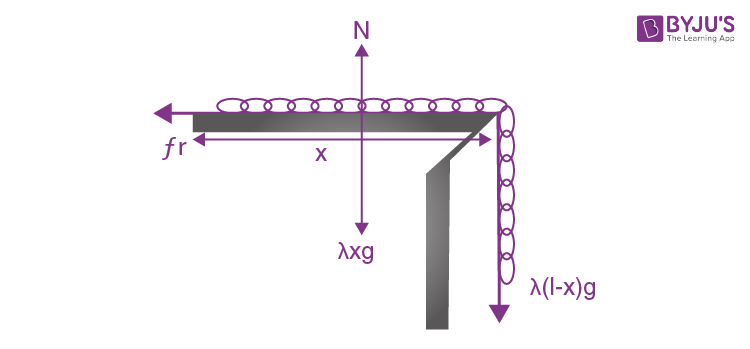Let (at an arbitrary moment of time) the length of the chain on the table is x. So the net friction force between the chain and the table, at this moment :

fr = kN = kλxg (2)

The differential work done by the friction forces:

dA =

$$\vec{f}_{r}.d\vec{r}$$

= -fr ds

= -kλxg(-dx)

= λg(η/(1-η))x dx (3)

Note that here we have written ds = -dx, because ds is essentially a positive term and as the length of the chain decreases with time, dx is negative)

Hence the sought work done

$$A = \int_{(1-\eta )l}^{0}\lambda g\frac{\eta }{1-\eta }x\: dx$$

= -(1-η)η mgl/2

= -1.3J

8. A body of mass m is thrown at an angle α to the horizontal with the initial velocity v0. Find the mean power developed by gravity over the whole time of motion of the body, and the instantaneous power of gravity as a function of time.

Solution:

1. The velocity of the body, t seconds after the beginning of the motion becomes

$$\vec{v}=\vec{v}_{0}+g\vec{t}$$

The power developed by the gravity

$$m\vec{g}$$

at that moment, is

$$P= m\vec{g}.\vec{v}$$

= mg(gt - v0 sin α) (1)

As

$$m\vec{g}$$
is a constant force, so the average power.

P = A/T =

$$\frac{m \vec{g}.\Delta \vec{r}}{T}$$

Where

$$\Delta \vec{r}$$
is the net displacement of the body during time of flight.

As,

$$m\vec{g}$$
perpendicular
$$\Delta \vec{r}$$

So P = 0

9. A particle of mass m moves along a circle of radius R with a normal acceleration varying with time as wn = at2, where a is a constant. Find the time dependence of the power developed by all the forces acting on the particle, and the mean value of this power averaged over the first t seconds after the beginning of motion.

Solution:

1. We have wn = v2/R = at2 or v = √(aR)t

t is defined to start from the beginning of motion from rest.

Instantaneous power,

$$P=\vec{F}.\vec{v}$$

=

$$m(w_{t}\hat{u_{t}}+w_{n}\hat{u_{t}}).(\sqrt{aR}t\hat{u_{t}})$$

Where

$$\hat{u_{t}}$$
and
$$\hat{u_{t}}$$
are unit vectors along the direction of tangent (velocity) and normal respectively)

So P = mwt√(aR)t = maRt

Hence the sought average power

$$P=\frac{\int_{0}^{t}P\: dt}{\int_{0}^{t}dt}=\frac{\int_{0}^{t}maRt\; dt}{t}$$

P = maRt2/2t = maRt/2

10. A small body of mass m is located on a horizontal plane at point O. The body acquires a horizontal velocity v0. Find:

1. (a) the mean power developed by the friction force during the whole time of motion, if the friction coefficient k = 0.27, m = 1.0 kg, and v0 = 1.5 m/s;

2. (b) the maximum instantaneous power developed by the friction force, if the friction coefficient varies as k = αx, where α is a constant, and x is the distance from the point O.

Solution:

1. Let the body m acquire the horizontal velocity v0 along positive x-axis at the point O.

(a) Velocity of the body t seconds after the beginning of the motion,

$$\vec{v}=\vec{v}_{0}+\vec{w}t$$

=

$$(v_{0}-kgt)\vec{i}$$

Instantaneous power P =

$$\vec{F}.\vec{v}$$

= -kmg(v0 - kgt)

From Eq. (1), the time of motion T = v0/kg

Hence sought average power during the time of motion

$$P=\frac{\int_{0}^{T}-kmg(v_{0}-kgt)dt}{T}$$

= -kmgv0/2

= -2W (on substitution)

From Fx = mwx

-kmg = mwx = mvxdvx/dx

Or vxdvx = -kgdx

= -αgx dx

To find v(x), let us integrate the above equation

$$\int_{v_{0}}^{v}v_{x}dv_{x}=-\alpha g\int_{0}^{x}x\: dx$$

Or v2 = v02 - αgx2 ...(1)

$$\vec{P}=\vec{F}.\vec{v}$$

= -mαxg√(v02 - αgx2) ..(2)

For maximum power, (d/dt)(√(v02x2 - λgx4) = 0 which yields x = v0/√(2αg)

Putting this value of x, in Eq. (2) we get,

Pmax = -½ mv02√(αg)

11. A small body of mass m = 0.10 kg moves in the reference frame rotating about a stationary axis with a constant angular velocity ω = 5.0 rad/s. What work does the centrifugal force of inertia perform during the transfer of this body along an arbitrary path from point 1 to point 2 which are located at the distances r1 = 30 cm and r2 = 50 cm from the rotation axis?

Solution:

1. Centrifugal force of inertia is directed outward along radial line, thus the sought work.

A =

$$\int_{r_{1}}^{r_{2}}m\omega ^{2}r\: dr$$

= ½ mω2(r22 - r12)

= 0.20 T (on substitution)

12. A system consists of two springs connected in series and having the stiffness coefficients k1 and k2. Find the minimum work to be performed in order to stretch this system by ∆l.

Solution:

1. Since the springs are connected in series, the combination may be treated as a single spring of spring constant.

K = K1K2/(K1 + K2)

From the equation of increment of M.E, ∆T + ∆U = Aext

0 + ½ k∆l2 = A

Or A = ½ (K1K2/(K1 + K2))∆l2

13. A body of mass m is hauled from the Earth's surface by applying a force F varying with the height of ascent y as F = 2 (ay - 1) mg, where a is a positive constant. Find the work performed by this force and the increment of the body's potential energy in the gravitational field of the Earth over the first half of the ascent.

Solution:

1. First, let us find the total height of ascent. At the beginning and the end of the path of the velocity of the body is equal to zero, and therefore the increment of the kinetic energy of the body is also equal to zero. On the other hand, according to the work-energy theorem, ∆T is equal to the algebraic sum of the works A performed by all the forces, i.e. by the force F and gravity, over this path. However, since ∆T = 0 then A = 0. Taking into account that the upward direction is assumed to coincide with the positive direction of the y-axis, we can write

$$A=\int_{0}^{h}(\vec{F}+m\vec{g}).d\vec{r}=\int_{0}^{h}(F_{y}-mg)dy$$

=

$$mg\int_{0}^{h}(1-2ay)dy$$

= mgh(1-ah)

= 0 where h = 1/a.

The work performed by the force F over the first half of the ascent is

Af =

$$\int_{0}^{\frac{h}{2}}F_{y}dy= 2mg\int_{0}^{\frac{h}{2}}(1-ay)dy$$

= 3mg/4a

The corresponding increment of the potential energy is

∆U = mgh/2 = mg/2a

14. The potential energy of a particle in a certain field has the form U = a/r2- b/r, where a and b are positive constants, r is the distance from the centre of the field. Find:

1. (a) the value of r0 corresponding to the equilibrium position of the particle; examine whether this position is steady;

2. (b) the maximum magnitude of the attraction force; draw the plots U (r) and Fr (r) (the projections of the force on the radius vector r).

Solution:

1. From the equation Fr = -dU/dr we get Fr = [-2a/r3 + b/r2]

(a) we have at r = r0, the particle is in equilibrium position. i.e.Fr = 0 so, r0 = 2a/b.

To check, whether the position is steady (the position of stable equilibrium), we have to satisfy

d2U/dr2 >0

We have d2U/dr2 = [6a/r4 - 2b/r3]

Putting the value of r = r0 = 2a/b, we get

d2U/dr2 == b4/8a3, (as a and b are positive constant)

So d2U/dr2 = b2/8a3 > 0

which indicates that the potential energy of the system is minimum, hence this position is steady.

(b) we have Fr = -dU/dr = [-2a/r3 + b/r2]

For F, to be maximum, dFr/dr = 0

So r = 3a/b and then Fr(max) = -b3/27a2

As Fr is negative, the force is attractive.

15. In a certain two-dimensional field of force the potential energy of a particle has the form U = αx2 + βy2 , where α and β are positive constants whose magnitudes are different. Find out:

1. (a) whether this field is central;

2. (b) what is the shape of the equipotential surfaces and also of the surfaces for which the magnitude of the vector of force F = const.

Solution:

1. (a) We have Fx = ∂U/dx = -2αx and Fy = -∂U/dy = -2βy

So

$$\vec{F}= 2\alpha x\vec{i}-2\beta y\vec{i}$$

And Fy = 2√(α2x2 + β2y2)

For a central force

$$\vec{r}\times \vec{F}=0$$

Here

$$\vec{r}\times \vec{F}=(x\vec{i}+y\vec{j})\times (-2\alpha x\vec{i}-2\beta y\vec{j})$$

=

$$-2\beta xy\vec{k}-2\alpha xy\vec{k}$$

≠ 0

Hence the force is not a central force.

(b) As U = αx2 + βy2

So, Fx = ∂U/∂x = -2αx and Fy = -∂U/∂y = -2βy

So F = √(Fx2 + Fy2)

= √(4α2x2 + 4β2y2)

According to the problem

F = 2 √(α2x2 + β2y2) = C (constant)

Or (α2x2 + β2y2) = C2/2

Or x22 + y22 = C2/2α2β2 = k (say)

Therefore the surfaces for which F is constant is an ellipse.

For an equipotential surface U is constant.

So αx2 + βy2 = C0 (constant)

Or x2/√β2 + y2/√α2 = C0/αβ = K0 (constant)

Hence the equipotential surface is also an ellipse.

16. There are two stationary fields of force F = ayi and F = axi + byj, where i and j are the unit vectors of the x and y axes, and a and b are constants. Find out whether these fields are potential.

Solution:

1. Let us calculate the work performed by the forces of each field over the path from a certain point 1 (x1, y1) to another certain point 2 (x2 , y2).

(i) dA =

$$\vec{F}.d\vec{r}= ay\vec{i}.d\vec{r}=ay \: dx$$

Or A =

$$a\int_{x_{1}}^{x_{2}}y\: dx$$

(ii) dA =

$$\vec{F}.d\vec{r}= (ax\vec{i}+by\vec{j}).d\vec{r}=ax\: dx+by\: dy$$

Hence A =

$$\int_{x_{1}}^{x_{2}}ax\: dx+\int_{y_{1}}^{y_{2}}by\: dy$$

In the first case, the integral depends on the function of type y (x), i.e. on the shape of the path. Consequently, the first field of force is not potential. In the second case, both the integrals do not depend on the shape of the path. They are defined only by the coordinate of the initial and final points of the path, therefore the second field of force is potential.

17. A body of mass in is pushed with the initial velocity v0 up an inclined plane set at an angle α to the horizontal. The friction coefficient is equal to k. What distance will the body cover before it stops and what work do the friction forces perform over this distance?

Solution:

1. Let s be the sought distance, then from the equation of increment of M.E.

∆T + ∆U = Afr

(0 - ½ mv02) + (mg s sin α) = -kmg cos α s

Or s = (v02/2g)/(sin α + k cos α)

Hence Afr = -kmg cos α s

= -kmv02/2(k + tan α)

18. A small disc A slides down with initial velocity equal to zero from the top of a smooth hill of height H having a horizontal portion (Fig. 1.30). What must be the height of the horizontal portion h to ensure the maximum distance s covered by the disc? What is it equal to?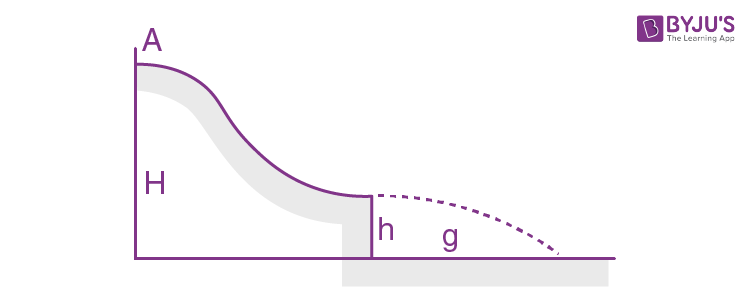Solution:

1. Velocity of the body at height h, vh = √(2g(H - h), horizontally (from the figure given in the problem). Time taken in falling through the distance h.

t = √(2h/g) (as initial vertical component of the velocity is zero.)

Now s = vht = √(2g(H + h)) ×√(2h/g)

= √(4(Hh - h2))

For smax, (d/ds)(Hh - h2) = 0, which yields h = H/2

Putting this value of h in the expression obtained for s, we get

smax = H

19. A small body A starts sliding from the height h down an inclined groove passing into a half-circle of radius h/2 (Fig. 1.31). Assuming the friction to be negligible, find the velocity of the body at the highest point of its trajectory (after breaking off the groove).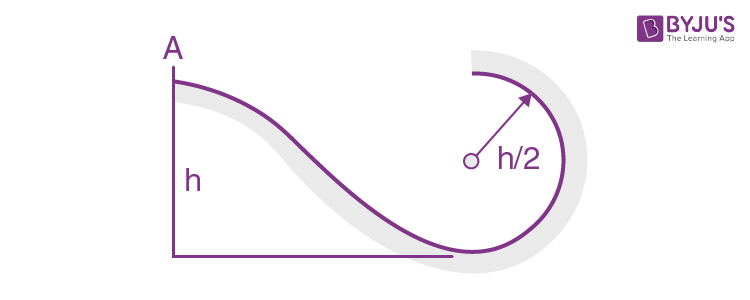Solution:

1. To complete a smooth vertical track of radius R, the minimum height at which a particle starts, must be equal to 5/2 R (one can prove it from energy conservation). Thus in our problem body could not reach the upper most point of the vertical track of radius R/2. Let the particle A leave the track at some point O with speed v (Fig.). Now from energy conservation for the body A in the field of gravity :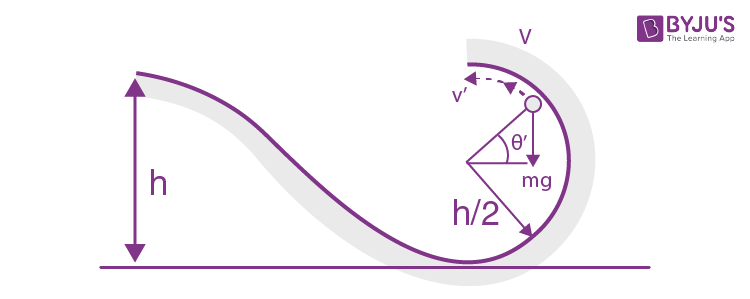mg(h - h(1 + sin θ)/2) = ½ mv2

Or v2 = gh(1 - sin θ) ..(1)

From Newton's second law for the particle at the point O; Fn = mwn,

N + mg sin θ = mv2/(h/2)

But, at the point O the normal reaction N = 0

So v2 = (gh/2) sin θ (2)

From (3) and (4), sin θ = ⅔ and v = √(gh/3)

After leaving the track at O, the particle A comes in air and further goes up and at maximum height of its trajectory in air, it’s velocity (say v') becomes horizontal (Fig.). Hence, the sought velocity of A at this point.

v’ = v cos (90 - θ) = v sin θ = ⅔ √(gh/3)

20. A ball of mass m is suspended by a thread of length l. With what minimum velocity has the point of suspension to be shifted in the horizontal direction for the ball to move along the circle about that point? What will be the tension of the thread at the moment it will be passing the horizontal position?

Solution:

1. Let the point of suspension be shifted with velocity vA in the horizontal direction towards left then in the rest frame of point of suspension the ball starts with same velocity horizontally towards right Let us work in this frame. From Newton’s second law in projection form towards the point of suspension at the upper most point (say B) :

mg + T = mvB2/l

Or T = mvB2/l - mg ..(1)

Condition required to complete the vertical circle is that T ≥ 0. But (2)

½ mvA2 = mg(2l) + ½ mvB2

So vB2 = vA2 - 4gl ..(3)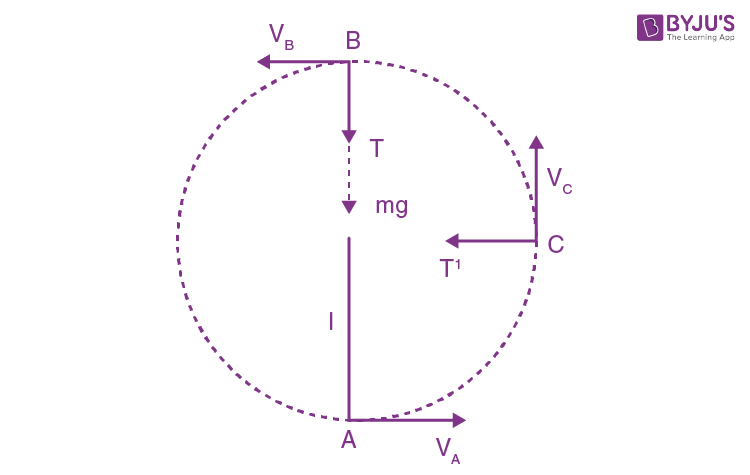From (1), (2) and (3)

T = m(vA2 - 4gl)/l - mg ≥ 0

Or vA ≥ √(5gl)

From the equation Fn = mwn at point C

T’ = mvc2/l ..(4)

Again from energy conservation

½ mvA2 = ½ mvc2 + mgl ..(5)

From (4) and (5)

T = 3mg